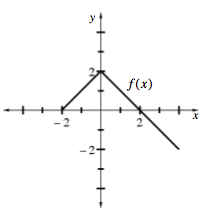### Home > CALC > Chapter 8 > Lesson 8.1.4 > Problem8-46

8-46.

Given f(x) at right, find values of k that will make each integral expression equivalent to $\int _ { 0 } ^ { 2 } f ( x ) d x$.

1. $k \int _ { - 2 } ^ { 2 } f ( x ) d x$

1. $k \int _ { - 2 } ^ { 4 } f ( x ) d x$

1. $k \int _ { 2 } ^ { 4 } f ( x ) d x$

1. $k \int _ { 4 } ^ { 2 } f ( x ) d x$

These answers are in no particular order.
Match the answer to the problem: